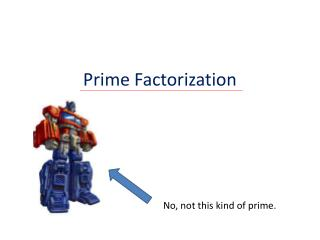DownloadDownload PresentationPrime Factorization

Prime Factorization

Download PresentationPrime Factorization

- - - - - - - - - - - - - - - - - - - - - - - - - - - E N D - - - - - - - - - - - - - - - - - - - - - - - - - - -
Presentation Transcript

1. Prime Factorization No, not this kind of prime.

2. What is a prime? • A prime is a natural number greater than 1 that has no positive divisors other than 1 and itself. • In other words, see what you can divide the • number by. • If the only answers are the number itself • and one, then the number is prime.

3. And if it’s not prime? If a number is not prime it is called composite. 6 is divisible by 1, 2, 3, and 6. This means that 6 is a composite number.

4. So what is prime factorization? • This is a way to break a number down into parts where each part is a prime number.

5. Let’s look at the number 6. 6 can be broken down into 3 x 2 6 3 x 2

6. For 6 we write the prime factorization as • 6 = 3 x 2

7. How do we find the prime factorization? • Division by primes • Factor Tree

8. Division by Primes • The first method is called division by primes (or the birthday cake method) • Let’s use the number 24 • First we pick a prime that we can divide 24 by. Since it is even we will try 2.

9. First we divide 24 by 2 and the result is 12 • Next we choose another prime to now divide 12 by • We divide 12 by 2 and the result is 6 • Now we choose a prime to divide 6 by, let’s choose 3 this time • The result is 2, which is a prime, once a prime answer is reached we stop • Now to get our answer we look on the outside of the long division 2 3 6 2 12 2 24 • The prime factorization of 24 is 2 x 2 x 2 x 3

10. The advantages to this method are in the ease of choosing your numbers. You must choose a prime number to divide by each time, which limits your choices to a few small numbers usually. The reason this is sometimes called the birthday cake method is because of the resulting shape. 2 3 6 2 12 2 24

11. Factor Tree • The second method is called a factor tree • Again we will use the number 24 • This time we are not limited to our choice of numbers to divide by so we will pick 8

12. 24 • Next we look at 8. We can divide 8 by 4 to • get a result of 2. First we divide 24 by 8. The result is 3. i.e. 8 x 3 = 24 8 3 • Then we look at the 3. This is a prime so we • do not touch the 3. We will circle it to indicate • we are done with it. • The 4 can turn into 2 x 2. 4 2 • The 2 is prime so we circle it. • The last two 2’s are prime, so again we circle • them. 2 2 • Now look at all of our circled numbers. • This is our prime factorization. • 24 = 3 x 2 x 2 x 2

13. 24 The advantage to the factor tree method is the ability to choose from any number. (as long you can divide the original number evenly) 8 3 • This method is named for the resemblance the final results have to a pine tree. 4 2 2 2

14. Which method should we use? • Both division by primes and factor tree may be used in any case; however, one method may make the problem much easier. • Often it is best to use division by primes when it is difficult to see large numbers that can be used. • Factor tree is a good choice when it is easy to see a large number to divide by. For example, numbers ending in zero (divide by ten).